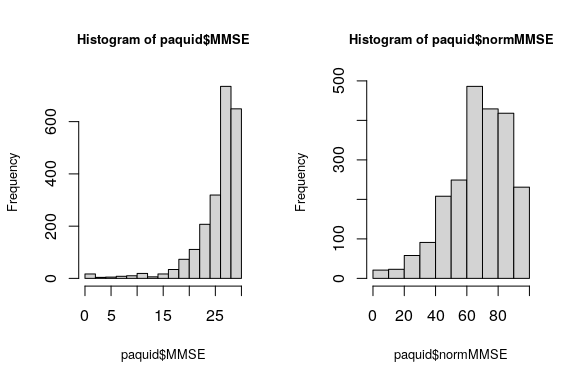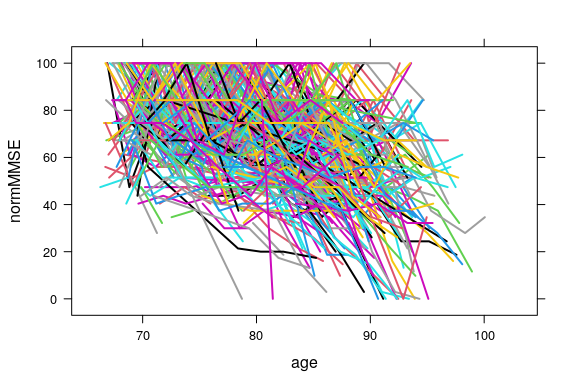# Functionalities of the package

The R package lcmm provides a series of functions to estimate statistical models based on the linear mixed model theory. It includes the estimation of:

• mixed models and latent class mixed models for Gaussian longitudinal outcomes (hlme),
• curvilinear and ordinal univariate longitudinal outcomes (lcmm)
• curvilinear multivariate outcomes (multlcmm)
• joint latent class mixed models (Jointlcmm) for a (Gaussian or curvilinear) longitudinal outcome and a time-to-event that can be possibly left-truncated right-censored and defined in a competing setting.

The package lcmm includes 4 main functions: lcmm, hlme, multlcmm and Jointlcmm. Each function is described in a different vignette. These functions apply to longitudinal markers, even those that have limited metrological properties but the functions can also be used in cross-sectional context.

For each model, maximum likelihood estimators are obtained using a modified Marquardt algorithm with strict convergence criteria based on the parameters and likelihood stability, and on the negativity of the second derivatives. The package also provides various post-fit functions including goodness-of-fit analyses, classification, plots, predicted trajectories, individual dynamic prediction of the event and predictive accuracy assessment.

# Calls functions

Each function is precisely introduced in the companion paper (Proust-Lima, JSS 2017 - https://doi.org/10.18637/jss.v078.i02).

## hlme

This function is used for standard linear mixed models and their extension to multiple profiles of trajectory : the latent class linear mixed models

hlme(fixed, mixture, random, subject, classmb, ng = 1, idiag = FALSE, nwg = FALSE, cor = NULL, data, B, convB = 0.0001, convL = 0.0001, convG = 0.0001, prior, maxiter = 500, subset = NULL, na.action = 1, posfix = NULL)

## lcmm

This function is used to estimate mixed models when the outcome does not have a Gaussian distribution. The method is called “latent process model”. As hlme, the method also handles heterogeneous profiles of trajectories in a “latent process and latent class mixed model”.

lcmm(fixed, mixture, random, subject, classmb, ng = 1, idiag = FALSE, nwg = FALSE, link = "linear", intnodes = NULL, epsY = 0.5, cor = NULL, data, B, convB = 1e-04, convL = 1e-04, convG = 1e-04,  maxiter = 100, nsim = 100, prior, range = NULL, subset = NULL, na.action = 1, posfix = NULL,  partialH = FALSE)

## multlcmm

This function extends lcmm function when more than one marker measures the same underlying construct of interest. Again the function handles either homogeneous or heterogeneous profiles of trajectories.

multlcmm(fixed, mixture, random, subject, classmb, ng = 1, idiag = FALSE, nwg = FALSE, randomY = FALSE, link = "linear", intnodes = NULL, epsY = 0.5, cor = NULL, data, B, convB = 1e-04, convL = 1e-04, convG = 1e-04, maxiter = 100, nsim = 100, prior, range = NULL, subset = NULL, na.action = 1, posfix = NULL, partialH = FALSE)

## Jointlcmm

This function extends hlme, lcmm and multlcmm to jointly analyze times to event (in a competing setting). The latent structure shared between the longitudinal outcome(s) and the time(s) to event is latent classes.

Jointlcmm(fixed, mixture, random, subject, classmb, ng = 1, idiag = FALSE, nwg = FALSE, survival, hazard = "Weibull", hazardtype = "Specific", hazardnodes = NULL, TimeDepVar = NULL, link = NULL, intnodes = NULL, epsY = 0.5, range = NULL, cor = NULL, data, B, convB = 1e-4, convL = 1e-4, convG = 1e-4, maxiter = 100, nsim = 100, prior, logscale = FALSE, subset = NULL, na.action = 1, posfix = NULL, partialH = FALSE)

# Package installation

If the package lcmm is not installed, install it:

install.packages("lcmm")

Each time you will open a R session, you will first have to load the lcmm library:

library(lcmm)

# Dataset example

## paquid subsample

In the wikis, lcmm is illutrated with paquid dataset. This is a subsample of 500 subjects from the original French prospective study Paquid. This dataset can not be used for epidemiological purposes as the subsample is not representative of the original cohort (in particular, dementia cases have been over sampled).

The data are in the longitudinal format including some variables such as 3 psychometric tests $$MMSE$$, $$BVRT$$, $$IST$$, the depressive symptomatology scale $$CESD$$ and the variables $$age$$, $$agedem$$ (age until dementia), $$dem$$ (boolean with 1 for dementia), $$ageinit$$, $$CEP$$ (education level) and $$male$$.

For visualizing the data (head of the table only):

head(paquid)
ID MMSE BVRT IST HIER CESD      age  agedem dem age_init CEP male
1  1   26   10  37    2   11 68.50630 68.5063   0  67.4167   1    1
2  2   26   13  25    1   10 66.99540 85.6167   1  65.9167   1    0
3  2   28   13  28    1   15 69.09530 85.6167   1  65.9167   1    0
4  2   25   12  23    1   18 73.80720 85.6167   1  65.9167   1    0
5  2   24   13  16    3   22 84.14237 85.6167   1  65.9167   1    0
6  2   22    9  15    3   NA 87.09103 85.6167   1  65.9167   1    0

The different markers are collected at different times. In the dataset the timescale is $$age$$.

For obtaining a quick summary of the data:

summary(paquid)
ID             MMSE            BVRT            IST
Min.   :  1.0   Min.   : 0.00   Min.   : 0.00   Min.   : 5.00
1st Qu.:132.2   1st Qu.:25.00   1st Qu.: 9.00   1st Qu.:22.00
Median :263.0   Median :27.00   Median :11.00   Median :27.00
Mean   :256.2   Mean   :25.99   Mean   :10.78   Mean   :26.52
3rd Qu.:376.0   3rd Qu.:29.00   3rd Qu.:13.00   3rd Qu.:31.00
Max.   :500.0   Max.   :30.00   Max.   :15.00   Max.   :40.00
NA's   :36      NA's   :300     NA's   :198
HIER            CESD             age             agedem
Min.   :0.000   Min.   : 0.000   Min.   : 66.28   Min.   :66.87
1st Qu.:1.000   1st Qu.: 2.000   1st Qu.: 75.09   1st Qu.:82.03
Median :1.000   Median : 6.000   Median : 80.57   Median :86.61
Mean   :1.272   Mean   : 8.488   Mean   : 80.53   Mean   :85.89
3rd Qu.:2.000   3rd Qu.:12.000   3rd Qu.: 85.77   3rd Qu.:89.94
Max.   :3.000   Max.   :52.000   Max.   :102.16   Max.   :99.49
NA's   :46      NA's   :146
dem            age_init          CEP              male
Min.   :0.0000   Min.   :65.25   Min.   :0.0000   Min.   :0.0000
1st Qu.:0.0000   1st Qu.:68.00   1st Qu.:0.0000   1st Qu.:0.0000
Median :0.0000   Median :71.92   Median :1.0000   Median :0.0000
Mean   :0.3329   Mean   :73.01   Mean   :0.7373   Mean   :0.3853
3rd Qu.:1.0000   3rd Qu.:76.96   3rd Qu.:1.0000   3rd Qu.:1.0000
Max.   :1.0000   Max.   :92.33   Max.   :1.0000   Max.   :1.0000


Some variables have missing values. This is not a problem when using lcmm package as missing values are systematically removed.

## MMSE outcome

In the lcmm examples, MMSE is usually considered as the outcome. MMSE is a very common neuropsychological test to measure global cognitive functioning in the elderly. It has a very asymmetric distribution so that it is usually normalized to be applied with methods for Gaussian variables. This is done using a pre-normalization function dedicated to MMSE provided in NormPsy package:

library(NormPsy)
paquid$normMMSE <- normMMSE(paquid$MMSE)
par(mfrow=c(1,2))
hist(paquid$MMSE, cex.main=0.8, cex.lab=0.8) hist(paquid$normMMSE, cex.main=0.8, cex.lab=0.8)The individual repeated measures of MMSE to be modelled are:

library(lattice)
color <- paquid\$ID
xyplot(normMMSE ~ age, paquid, groups = ID, col=color, lwd=2, type="l")